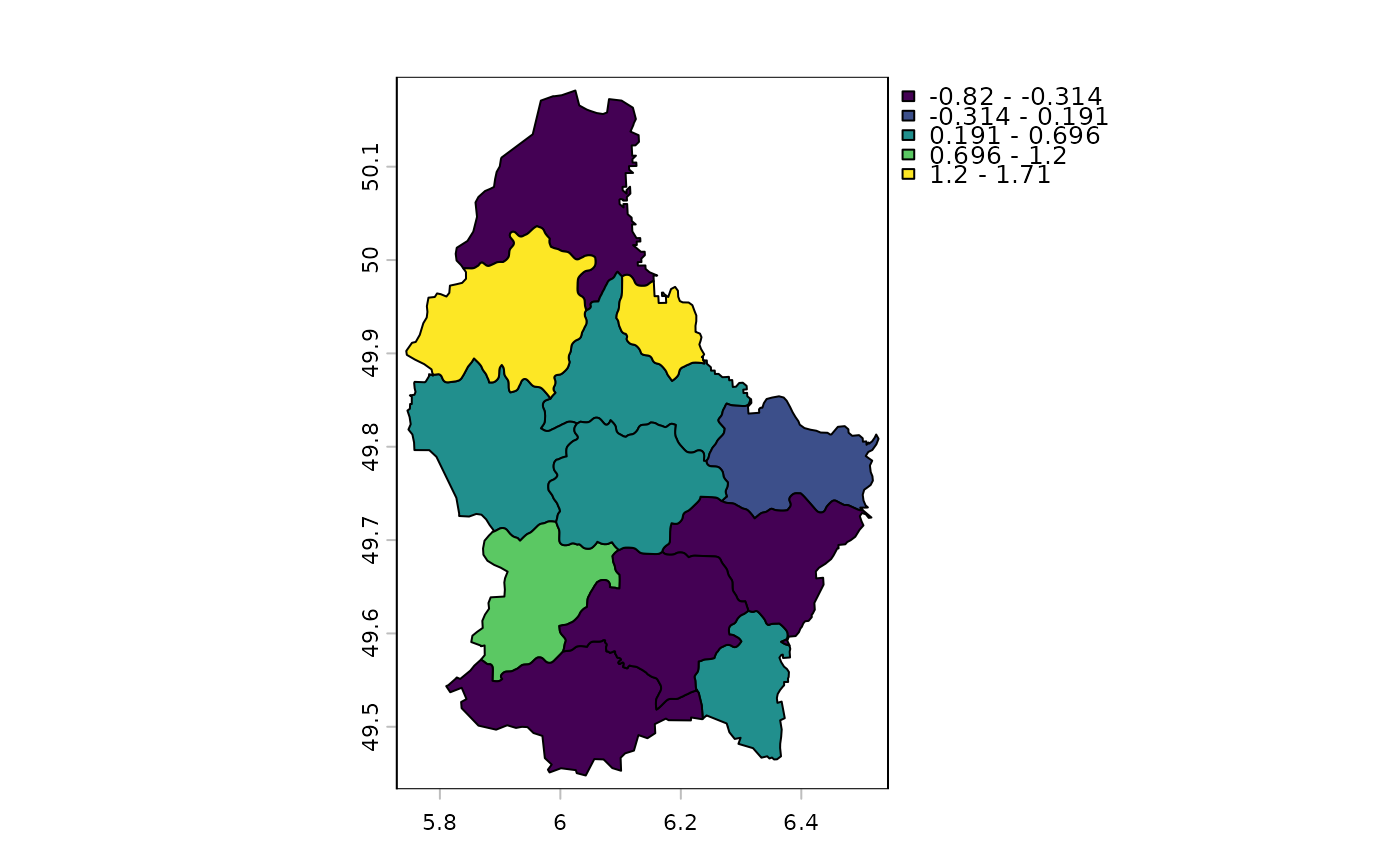Compute spatial autocorrelation for a numeric vector or a SpatRaster. You can compute standard (global) Moran's I or Geary's C, or local indicators of spatial autocorrelation (Anselin, 1995).

# S4 method for numeric
autocor(x, w, method="moran")

# S4 method for SpatRaster
autocor(x, w=matrix(c(1,1,1,1,0,1,1,1,1),3), method="moran", global=TRUE)

## Arguments

x

numeric or SpatRaster

w

Spatial weights defined by or a rectangular matrix. For a SpatRaster this matrix must the sides must have an odd length (3, 5, ...)

global

logical. If TRUE global autocorrelation is computed instead of local autocorrelation

method

character. If x is numeric or SpatRaster: "moran" for Moran's I and "geary" for Geary's C. If x is numeric also: "Gi", "Gi*" (the Getis-Ord statistics), locmor (local Moran's I) and "mean" (local mean)

## Value

numeric or SpatRaster

## Details

The default setting uses a 3x3 neighborhood to compute "Queen's case" indices. You can use a filter (weights matrix) to do other things, such as "Rook's case", or different lags.

The spdep package for additional and more general approaches for computing spatial autocorrelation

## Examples

### raster
r <- rast(nrows=10, ncols=10, xmin=0)
values(r) <- 1:ncell(r)

autocor(r)
#>    lyr.1
#> 1.117188

# rook's case neighbors
f <- matrix(c(0,1,0,1,0,1,0,1,0), nrow=3)
autocor(r, f)
#> lyr.1
#>  1.25

# local
rc <- autocor(r, w=f, global=FALSE)

### numeric (for vector data)
f <- system.file("ex/lux.shp", package="terra")
v <- vect(f)
w <- relate(v, relation="touches")

# global
autocor(v$AREA, w) #>  -0.1531971 # local v$Gi <- autocor(v\$AREA, w, "Gi")
plot(v, "Gi")